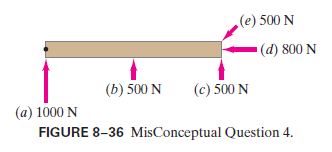# Problem: The solid dot shown in Fig. 8–36 is a pivot point. The board can rotate about the pivot. Which force shown exerts the largest magnitude torque on the board?

###### FREE Expert Solution

Torque is expressed as:

$\overline{)\begin{array}{rcl}{\mathbf{\tau }}& {\mathbf{=}}& \mathbf{r}\mathbf{×}\mathbf{F}\\ & {\mathbf{=}}& \mathbf{r}\mathbf{F}\mathbf{s}\mathbf{i}\mathbf{n}\mathbf{\theta }\end{array}}$, where r is the distance between the force and the pivot, F is the magnitude of the force, and θ is the angle between r and F.

(a)

F = 1000 N

r = 0 m

τ1000N = (0)(1000)sinθ = 0 m•N

80% (110 ratings)###### Problem Details

The solid dot shown in Fig. 8–36 is a pivot point. The board can rotate about the pivot. Which force shown exerts the largest magnitude torque on the board?